categories :: Markup-Test :: all

# Typesetting

Emphasized Text.

\SMALL, \Small, \small

\big, \Big, \BIG

\center

top to the very bottom

You can see in  and on ompf  that the truth is not necessarily out there 0

# Images

Images of picogen (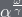). Fortunatley, thumbnails are automagically generated by invocation of some magick 3.

# LaTeX-formulas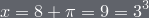.

Using math-symbols en masse in prime-time-television makes that appearance no way better. Take for example: 4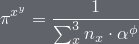. Doesn't that look astonishing? No. It does not.

Don't forget that you can click the formulas to get a bigger and printable version 5.

# Verbatim

It follows a part 6 of source markup that has been used to generate this site:

\date{2008-06-10}\title{Testpage}\category{Markup-Test}\class{markup-test}\maketitle{true}\section {Typesetting}{    \emph{Emphasized Text.}    \SMALL{\\SMALL}, \Small{\\Small}, \small{\\small}    \big{\\big}, \Big{\\Big}, \BIG{\\BIG}    \center{\\center}    \Big{\sup{\sup{top} to} the \sub{very \sub{bottom}}}    You can see in \reference{Preetham: A Practical Analytical Model ...} and on ompf 	\ref{\url{http://ompf.org/forum}, the ompf.org forum} that the truth	is not necessarily out there \footnote{You don't have to believe that it is not possible	to have ... \footnote{... footnodes inside other ... \footnote{... footnotes. 	proof:	\display-math{\pi = 2.9^{\phi\delta x}}. See? Madness.}}}}\section {Images}{    Images of picogen  (\math{\alpha^{\frac{\omega}{\gamma}}}).     Fortunatley, thumbnails are automagically generated by invocation of some magick     \footnote{\image{markup-test-images/49278848.png}}.        \center{        \image-s{416}{markup-test-images/picogen_0002.jpg}            \image-s{196}{markup-test-images/zbncubemap.png}        \image-s{196}{markup-test-images/sys-evac.png}            \image-s{416}{markup-test-images/picogen_0009.png}    }}\section {LaTeX-formulas}{	Let \math{x=3^3}, then \math{x=8+\pi}, with \math{\pi=1}, so that     \display-math{x=8+\pi=9=3^3}.            Using math-symbols en masse in prime-time-television makes that appearance no way better.     Take for example:      \footnote{        That is because             \display-math{\pi^{x^y}=\frac{1}{\sum^3_x n_x \cdot \alpha^\phi}}         really is without any sense.    }	\display-math{\pi^{x^y}=\frac{1}{\sum^3_x n_x \cdot \alpha^\phi}}.    Doesn't that look astonishing? No. It does not.         \emph{\big{Don't forget that you can click the formulas to get a bigger and printable version    \footnote{I will write a full PDF exporter soon}.}} }\section {Verbatim} {    \emph{        It follows a part \footnote{This article is not a quine        \reference{\url{http://en.wikipedia.org/wiki/Quine_(computing)}}}         of source markup that has been used to generate this site:    }    \code{        Not a quine!    }}    

# footnotes

 0: You don't have to believe that it is not possible to have ... 1 1: ... footnodes inside other ... 2 2: ... footnotes. proof: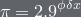. See? Madness. 3: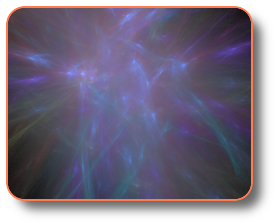4: That is becausereally is without any sense. 5: I will write a full PDF exporter soon 6: This article is not a quine

# references

 0: Preetham: A Practical Analytical Model ... 1: http://ompf.org/forum, the ompf.org forum 2: http://en.wikipedia.org/wiki/Quine_(computing)

top